We will continue our discussion of the Golden Ratio by discussing the Fibonacci and Lucas series here.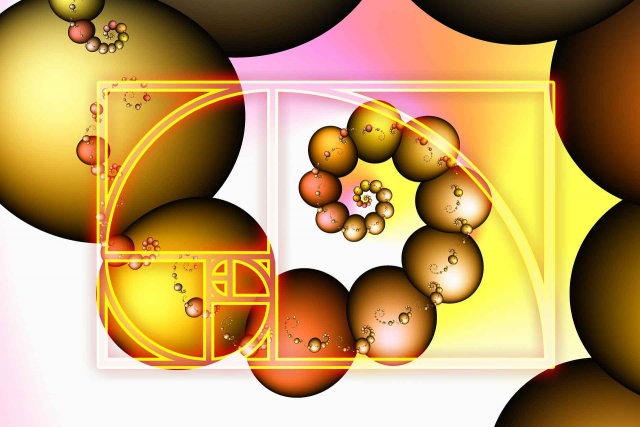Fibonacci Series

This series was popularized by Leonardo Fibonacci (Leonardo Filius Bonaccio 1175-1250) an Italian mathematician.

He introduced the Fibonacci Series to Europe and popularized the Hindu-Arabic number system (also called the decimal system).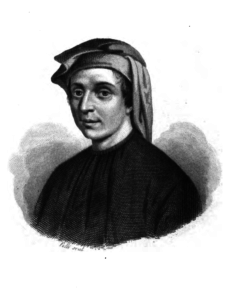The Fibonacci Series is a self-generating number series that is:

• additive – each number is the sum of the previous two
• multiplicative – each number approximates the previous number multiplied by the golden section

It begins with zero and unity, 0 & 1, nothing and everything, the Unknowable and the manifest Monad.

0, 1, 1, 2, 3, 5, 8, 13, 21, 34, 55, 89, 144, 233, 377…

The 12th term, divided into unity = 89/1 = .01123581321345589144…

Interestingly, this is an endless decimal that replicates the entire Fibonacci sequence.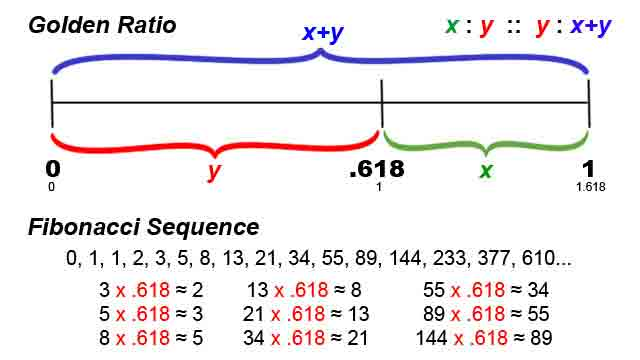“The series grows by accruing terms that come from within itself, from its immediate past, taking nothing from outside the sequence for its growth.”1  Much like the ouroboros: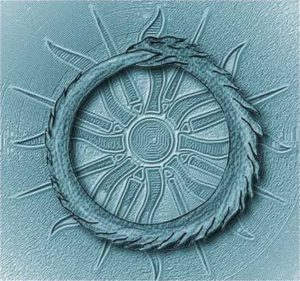Each number is the sum of the two preceding:

1 + 1 = 2

1 + 2 = 3

2 + 3 = 5

3 + 5 = 8

5 + 8 = 13

8 + 13 = 21…etc.

Fibonacci Ratios Appear:

• In the ratio of the number of spiral arms in daisies & sunflowers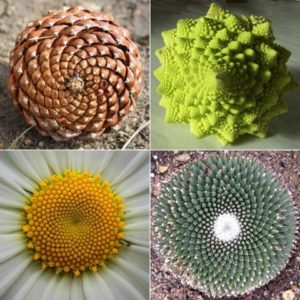• In the chronology of rabbit populations
• In the family tree of bees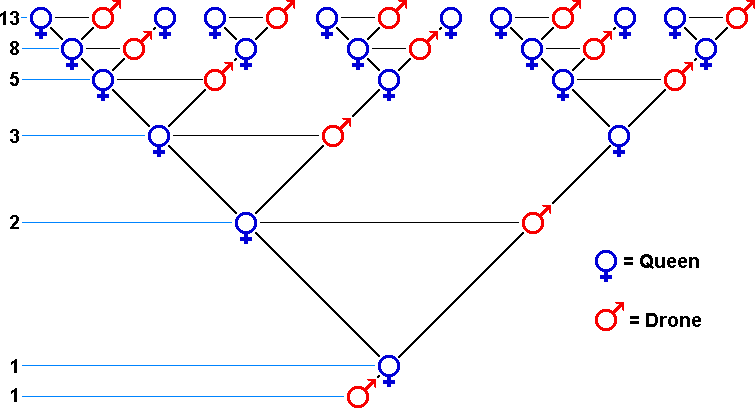• In the sequence of leaf patterns as they twist around a branch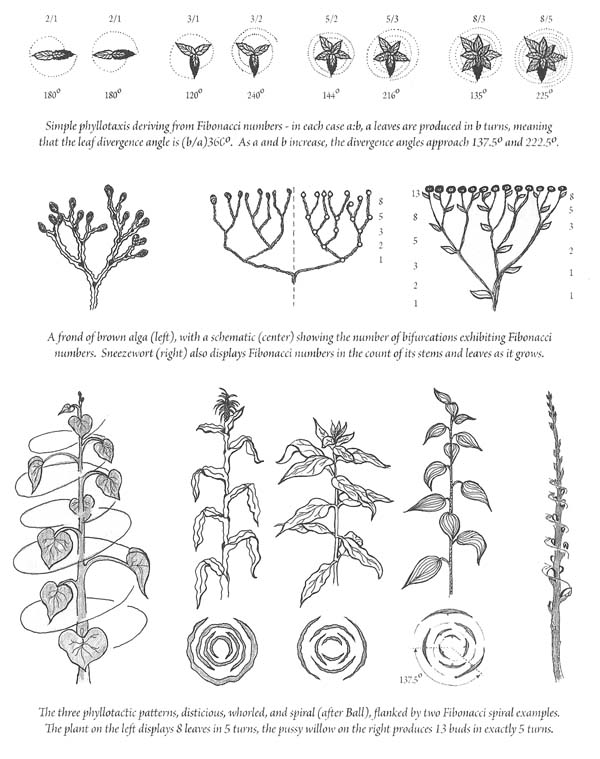Credit: Designa – page 311

• In the average number of petals on each type of flower in a field• In the numbers of pine needles accumulating as clusters in different pine species (3, 5 & 8)• In the spiraling bracts of pine cones and other seed structures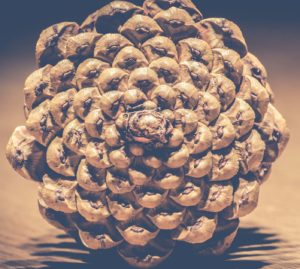• In the structure of the 12-note chromatic musical scale on a keyboard:
• 5 black keys
• 8 white keys
• 13 total keys making one full octave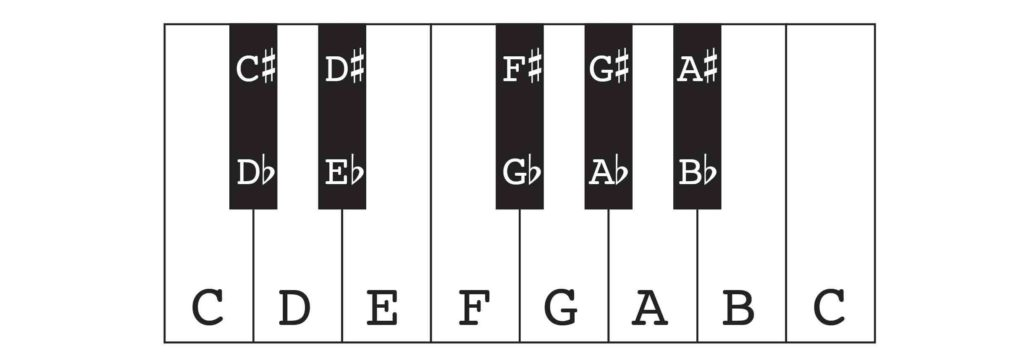• In stock market patterns
• In hurricane clouds• In self-organizing DNA nucleotides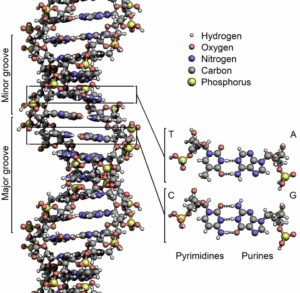• Throughout chemistry
• In uranium oxide compounds U2O5, U3O8, U5O13, U8O21, U13O34
• In many places in nature where self-generating patterns are in effect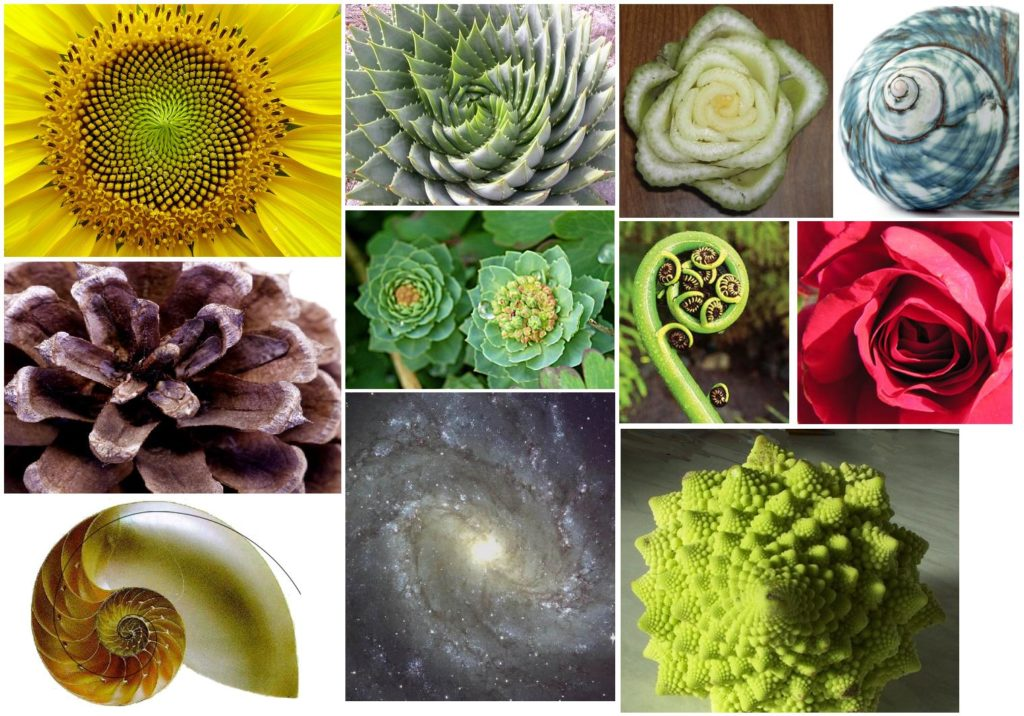“This principle of ongoing growth-from-within is the essence of the Pentad’s principle of regeneration and the pulsing rhythms of natural growth and dissolution.  It appears in plants, music, seashells, spiral galaxies, the human body, and everything associated with the fiveness in nature.”2

Fibonacci Sequence & Phi (the Golden Ratio)

The Fibonacci sequence is closely associated with the phi ratio – particularly the multiplicative aspect.Look at each number as a ratio of each successive pair:

• 1/1
• 2/1
• 3/2
• 5/3
• 8/5
• 13/8
• 21/13
• 34/21
• 55/34…etc.

Then take the decimal value of that ratio.  As a limit it approaches the golden section but it never gets there.

• 1/1 =1
• 2/1=2
• 3/2=1.5
• 5/3=1.6666
• 8/5=1.6
• 13/8=1.625
• 21/13=1.615384
• 34/21=1.619048…and so on

This is called asymptotically reaching a limit.

As seen below, these ratios bounce back and forth coming closer and closer to the phi ratio as you move up in the Fibonacci sequence.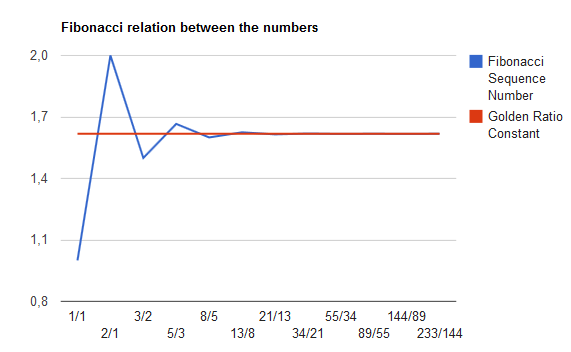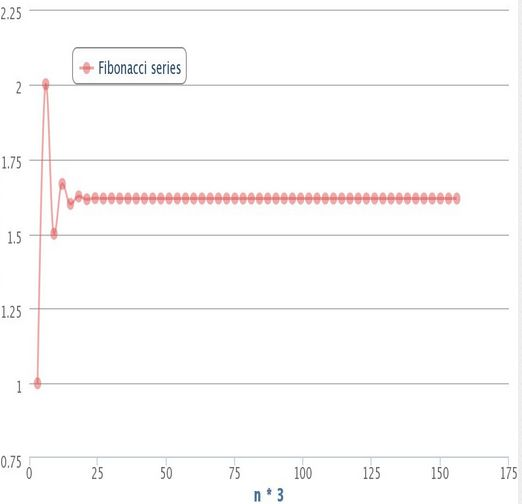This is nature’s way of dealing with infinities in the physical realm.

Phi is a ratio of adjacent terms of the Fibonacci Series evaluated at infinity.

“The sequence is the rational progression towards the irrational number embodied in the quintessential golden ratio.”3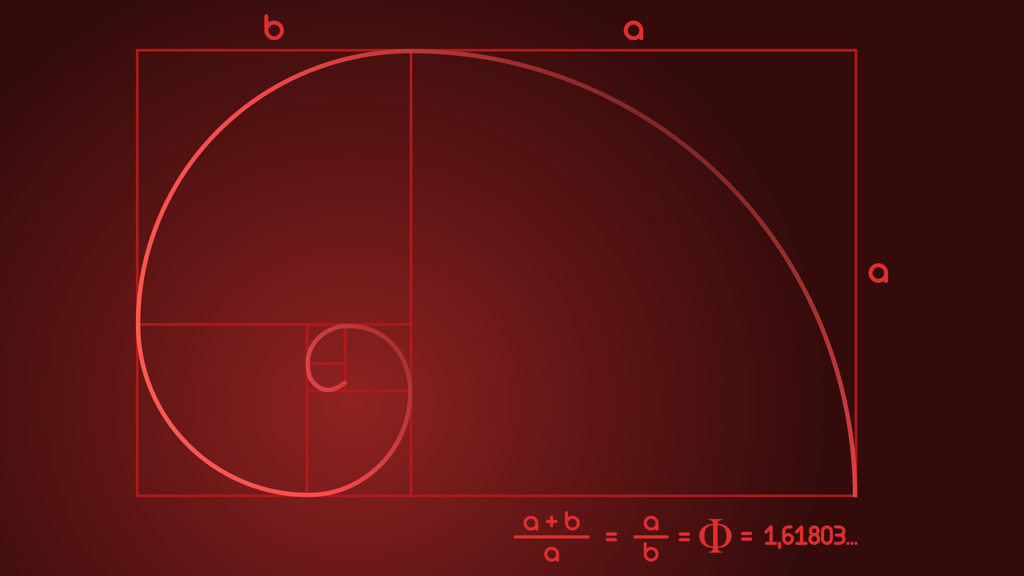You can actually select any 2 numbers, not just 0 & 1, adding each consecutive pair to get the next term and the ideal limit will always approach phi.

Different sequences become more exact at different rates.

The Fibonacci and Lucas series do it the quickest.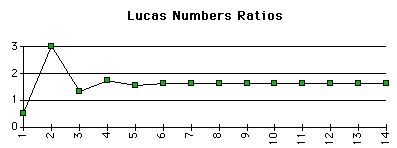Like Fibonacci numbers, Lucas numbers tend to phi.

“The Fibonacci sequence also has a pattern that repeats every 24 numbers.  This repetitive pattern involves a simple technique called numeric reduction in which all the digits of a number are added together until only 1 digit remains.  As an example, the numeric reduction of 256 is 4 because 2 + 5 + 6 = 13 and 1 + 3 = 4.  Applying numeric reduction to the Fibonacci series produces an infinite series of 24 repeating digits:

1, 1, 2, 3, 5, 8, 4, 3, 7, 1, 8, 9, 8, 8, 7, 6, 4, 1, 5, 6, 2, 8, 1, 9.”4

Lucas Number Series

The Lucas series is named after the French mathematician Edouard Lucas (1842-1891).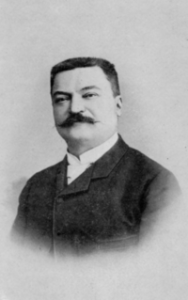Lucas numbers are similar to Fibonacci numbers.

They are:

• additive – each new number is the sum of the previous numbers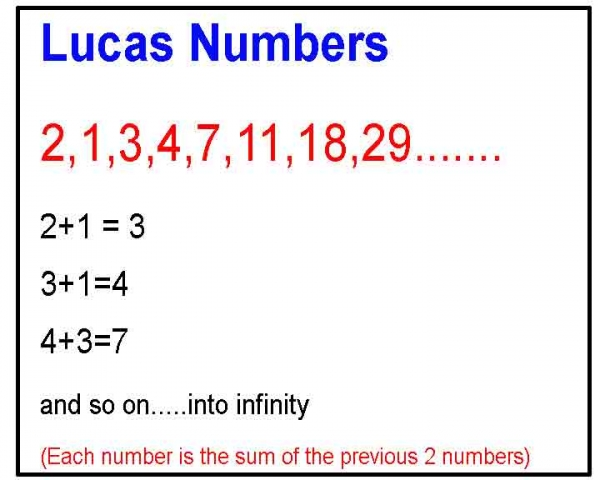• multiplicative – each new number approximates the previous number multiplied by phi
• Dividing any term in the sequence by the previous term approximates phi.

The sequence is:

2, 1, 3, 4, 7, 11, 18, 29, 47, 76, 123, 199…

The first four integers, the basis of the Tetraktys, are Lucas numbers (1, 2, 3, 4).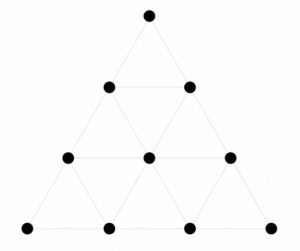The Tetraktys: 1, 2, 3, and 4 dots per row

The Lucas numbers are formed by alternately adding and subtracting the golden powers of phi and its reciprocal 1/phi.

The two irrational parts either zip together or peel apart to form the integers.

These are absolutely exact, and not approximations.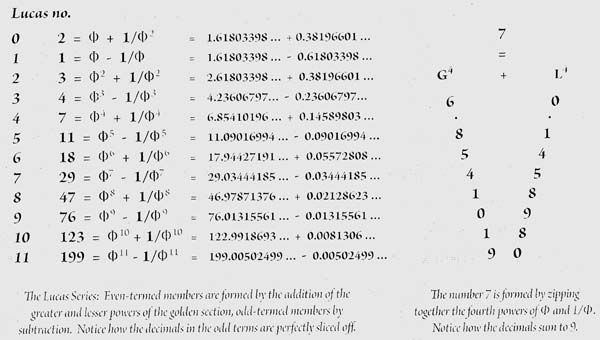Credit: Chart, page 313 in Designa.

Lucas numbers are also found in Nature.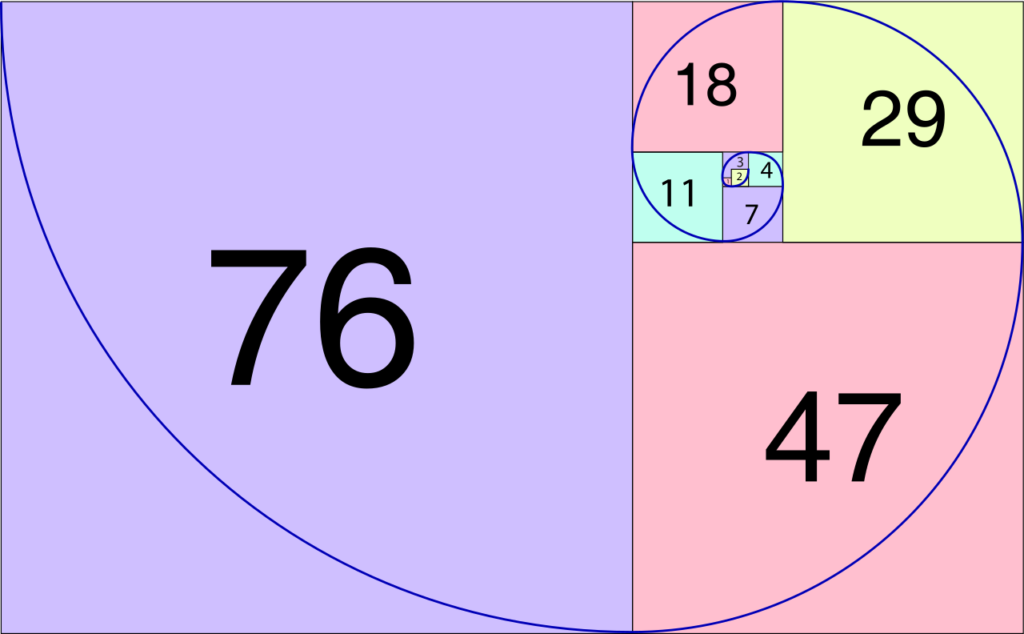The Lucas number spiral is a logarithmic spiral found in Nature.  It closely approximates the golden ratio when using larger terms.  The smaller terms used the farther it gets from phi.

The Lucas divergence angle = 99.5º.  This is 360/(1 + Φ2).

This is found in 1.5% of observed phyllotaxis plant patterns including:

some sunflowers, certain cedars, sequoias, balsam trees and other species.1. Schneider, Michael, A Beginner’s Guide to Constructing the Universe, Harper Perennial, 1994
2. ibid.
3. https://www.geometrycode.com/
4. Meisner, Gary B., The Golden Ratio: The Divine Beauty of Mathematics, Race Point Publishing, 2018